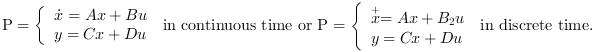Scilab Home page | Wiki | Bug tracker | Forge | Mailing list archives | ATOMS | File exchange
Scilab 6.0.1
Change language to: English - Français - 日本語 - Русский

See the recommended documentation of this function

# lqi

Linear quadratic integral compensator (full state)

### Syntax

`[K,X]=lqi(P,Q,R [,S])`

### Arguments

P

The plant state space representation (see syslin) with nx states, nu inputs and ny outputs.

Q

Real nx+ny by nx+ny symmetric matrix,

R

full rank nu by nu real symmetric matrix

S

real nx+ny by nu matrix, the default value is zeros(nx+ny,nu)

K

a real matrix, the optimal gain

X

a real symmetric matrix, the stabilizing solution of the Riccati equation

### Description

This function computes the linear quadratic integral full-state gain K for the plant P. The associated system block diagram is:The plant P is given by its state space representationThe cost function in l2-norm is:whereandis the integrator(s) state(s);

### Algorithm

The lqi function solves the lqr problem for the augmented plant### Caution

It is assumed that matrixis non singular.

### Remark

If the full state of the system is not available, An estimator of the plant state can be built using the lqe function.

### Examples

Linear quadratic integral controller of a simplified disk drive using state observer.```//Disk drive model
G=syslin("c",[0,32;-31.25,-0.4],[0;2.236068],[0.0698771,0]);
t=linspace(0,20,2000);
y=csim("step",t,G);

//State estimator
Wy=1;
Wu=1;
S=0;
Q=G.B*Wu*G.B';
R=Wy+G.D*S + S'*G.D+G.D*Wu*G.D';
S=G.B*Wu*G.D'+S;

//State estimator
[Kf,X]=lqe(G,Q,R,S);
Gx=observer(G,Kf);

//LQI compensator

wy=100;
Q= wy*sysdiag(G.C'*G.C,1);
R=1/wy;
Kc=lqi(G,Q,R);
//full controller
K=lft([1;1]*(-Kc(1:2)*Gx(:,[2 1])+Kc(3)*[1/%s 0]),1);//e-->u

//Full system
H=(-K*G)/.(1);// full system transfer function

y=csim("step",t,H);
clf;plot(t,y)```

• observer — observer design
• lqr — LQ compensator (full state)
• lqg — LQG compensator
• lft — linear fractional transformation